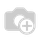# How to generate serial number and total quantity in qweb report without parser file ?

Hello Everyone,

I want to generate dynamic serial number and also generate total quantity without parser file.

here given example to more clarify.

serial no                                              product                                description                                quantity                                 Total

1                                                          any product                              -                                           2                                              -

2                                                               -                                          -                                            2                                              -

3                                                               -                                          -                                            2                                              -

?                                                               -                                          -                                            2                                              -

?                                                               -                                          -                                            2                                              -

?                                                               -                                          -                                            2                                              -

Total quantity                  ?(12)

we can generate serial number using parser file but I want generate serial number and total quantity without parser file

1 CommentDo you mean that you want to dynamically compute serial numbers and quantity in qweb?

You could try something like this for serial number:

`<t t-foreach="o.lines" t-as="p">  <span t-esc="p_index"></t> `

The "_index" is automatically understood by qweb to generate the index number

If you want to create your own, you could try something like:

`<t t-set="i" t-value="1" /> <t t-foreach="o.lines" t-as="p">  <span t-esc="i">   <t t-set="i" t-value="i+1"/> </t>`

EDIT:

For adding quantity dynamically, I assume your code to display quantity looks something like this:

`<t t-foreach="o.lines" t-as="p">  <span t-esc="p.quantity">  `

You need to initialize a variable before the loop, and simply add the quantity to it for each iteration:

`<t t-set="i" t-value="0" /> <t t-foreach="o.lines" t-as="p">  <span t-esc="i">   <t t-set="i" t-value="i+p.quantity"/> </t> <t t-esc="i" />`

This way you should get the quantity

Let me know if this doesn't workThanks @Shawn this code is very help for me to generate dynamically serial number in qweb report can you little help for how to generate dynamic total quantity ?

Thanks again @Shawn Your updated code is fully work.Now I get the total quantity dynamically without parser file.

Check this post

I write a more extensive explanation of qweb report parsers at:

https://www.odoo.com/es_ES/forum/help-1/question/how-to-define-a-custom-methods-functions-to-be-used-in-a-qweb-report-how-to-define-and-use-a-report-parser-92244thank a lot @Shawn Varghese